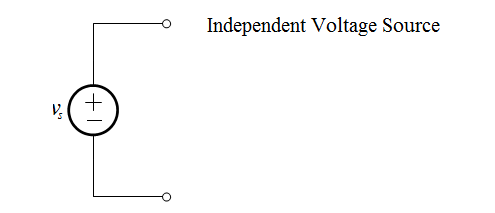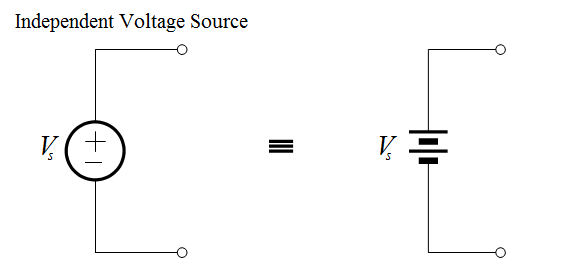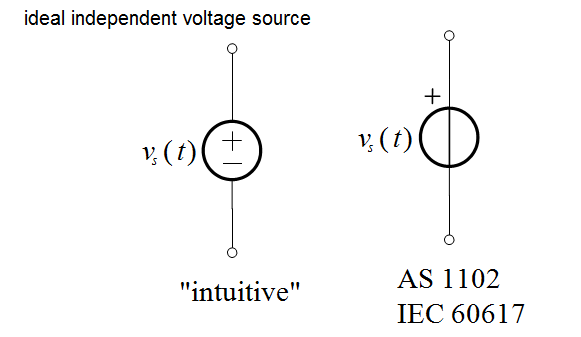# Circuit Elements and Types of Circuits

A  circuit  element  is  an  idealised  mathematical  model  of  a  two-terminal electrical device that is completely characterised by its voltage-current relationship. Although ideal circuit elements are not “off-the-shelf” circuit components, their importance lies in the fact that they can be interconnected (on paper or on a computer) to approximate actual circuits that are composed of nonideal elements and assorted electrical components – thus allowing for the analysis of such circuits.

Circuit elements can be categorized as either active or passive.

Active Circuit Elements

Active circuit elements can deliver a non-zero average power indefinitely. There are four types of active circuit element, and all of them are termed an ideal source. They are:

• Independent voltage source
• Independent current source
• Dependent voltage source
• Dependent current source

Passive Circuit Elements

Passive circuit elements cannot deliver a non-zero average power indefinitely. Some passive elements are capable of storing energy, and therefore delivering power back into a circuit at some later time, but they cannot do so indefinitely.

There are three types of passive circuit element. They are:

• Resistor
• Inductor
• Capacitor

Types of Circuits

The  interconnection  of  two  or  more  circuit  elements  forms  an  electrical network. If the network contains at least one closed path, it is also an electrical circuit. A network that contains at least one active element, i.e. an independent or dependent source, is an active network. A network that does not contain any active elements is a passive network.

Independent Sources

Independent sources are ideal circuit elements that possess a voltage or current value that is independent of the behaviour of the circuits to which they belong.

The Independent Voltage Source

An independent voltage source is characterised by a terminal voltage which is completely independent of the current through it. The representation of an independent voltage source is shown below:If the value of the voltage source is constant, that is, does not change with time, then we can also represent it as an ideal battery:Although a “real” battery is not ideal, there are many circumstances under which an ideal battery is a very good approximation.

In general, however, the voltage produced by an ideal voltage source will be a function of time. In this case we represent the voltage symbolically as v(t ) .

A few typical voltage waveforms are shown below. The waveforms in (a) and (b) are typical-looking amplitude modulation (AM) and frequency modulation (FM) signals, respectively. Both types of signals are used in consumer radio communications. The sinusoid shown in (c) has a wide variety of uses; for example, this is the shape of ordinary household voltage. A “pulse train”, such as that in (d), can be used to drive DC motors at a variable speed.Since the voltage produced by a source is in general a function of time, then the most general representation of an ideal voltage source is as shown below:The Independent Current Source

An independent current source establishes a current which is independent of the voltage across it. The representation of an independent current source is shown below:In other words, an ideal current source is a device that, when connected to anything, will always push current ( is) out of terminal 1 and pull is into terminal 2

Since the current produced by a source is in general a function of time, then the most general representation of an ideal current source is as shown below: